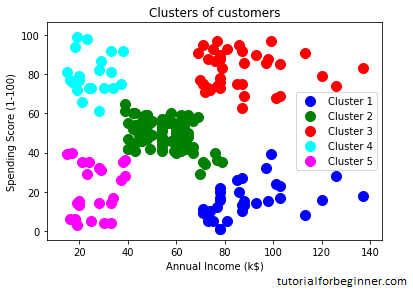# Hierarchical Clustering in Machine Learning

In this page, we will learn Hierarchical Clustering in Machine Learning, What is Hierarchical Clustering?, Why hierarchical clustering?, Agglomerative Hierarchical clustering, How the Agglomerative Hierarchical clustering Work?, Measure for the distance between two clusters, Woking of Dendrogram in Hierarchical clustering, Python Implementation of Agglomerative Hierarchical Clustering, Steps for implementation of AHC using Python.

## What is Hierarchical Clustering?

Hierarchical clustering, also known as hierarchical cluster analysis or HCA, is another unsupervised machine learning approach for grouping unlabeled datasets into clusters.

The hierarchy of clusters is developed in the form of a tree in this technique, and this tree-shaped structure is known as the dendrogram.

Although the results of K-means clustering and hierarchical clustering may appear to be comparable at times, their methods differ. As opposed to the K-Means algorithm, there is no need to predetermine the number of clusters.

There are two ways to hierarchical clustering:

• Agglomerative: It is a bottom-up strategy in which the algorithm starts with all data points as single clusters and merges them until only one cluster remains.
• Divisive: As a top-down method, the divisive algorithm is the polar opposite of the agglomerative algorithm.

## Why hierarchical clustering?

Why do we need hierarchical clustering when we already have alternative clustering algorithms like K-Means Clustering? So, as we've seen with K-means clustering, this algorithm has some limitations, such as a set number of clusters and a constant attempt to construct clusters of the same size. We can utilize the hierarchical clustering algorithm to tackle these two problems because we don't need to know the specified number of clusters in this algorithm.

The Agglomerative Hierarchical Clustering algorithm will be discussed in this topic.

## Agglomerative Hierarchical clustering

A notable example of HCA is the agglomerative hierarchical clustering algorithm. It uses a bottom-up strategy to group the datasets into clusters. It means that this algorithm treats each dataset as a single cluster at first, and then starts joining the clusters that are the closest to each other. This is repeated until all of the clusters have been merged into a single cluster containing all of the datasets.

The dendrogram is a diagram that depicts the hierarchy of clusters.

## How the Agglomerative Hierarchical clustering Work?

The following stages can be used to describe how the AHC algorithm works:

Step 1: Create a single cluster for each data point. Let's imagine there are N data points, which means there will be N clusters.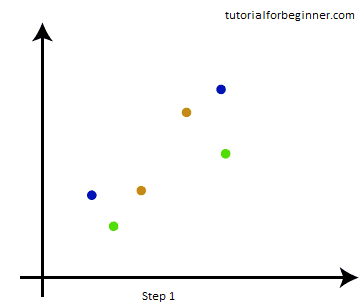Step-2: Merge the two nearest data points or clusters to form a single cluster. As a result, N-1 clusters will now exist.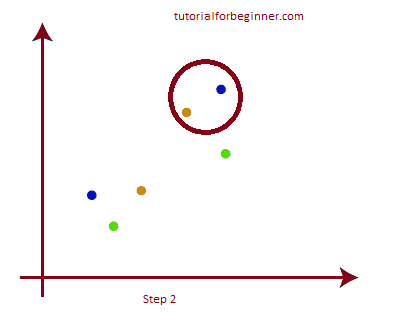Step-3: Combine the two nearest clusters once more to produce a single cluster. N-2 clusters will exist.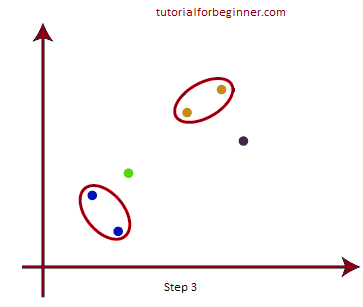Step-4: Continue with Step 3 until there is just one cluster left. As a result, we'll have the following clusters. Consider the following examples: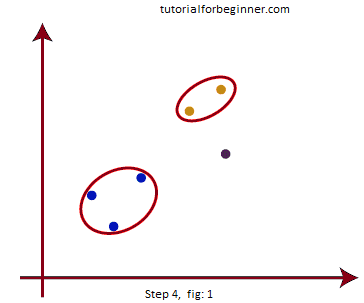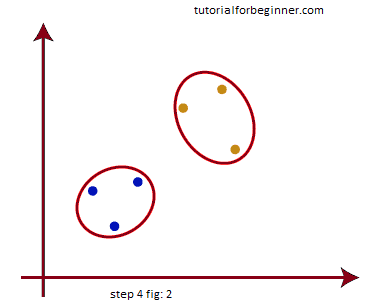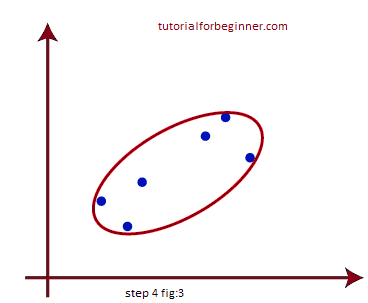Step-5: Create a dendrogram to separate the clusters according to the problem once all of the clusters have been joined into one large cluster.

## Measure for the distance between two clusters

The closest distance between the two groups, as we've seen, is critical for hierarchical clustering. There are several methods for calculating the distance between two clusters, and these methods determine the clustering rule. Linkage methods are the term for these kind of metrics. The following are some of the most popular connection methods:

Single Linkage: The shortest distance between the clusters' nearest locations. Consider the following illustration: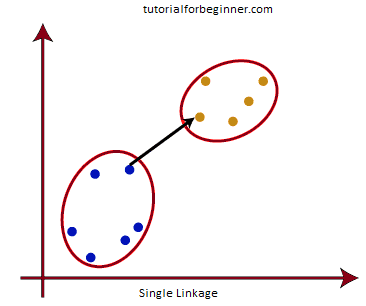Complete Linkage: It is the distance between the two points of two separate clusters that is the greatest. Because it creates tighter clusters than single-linkage, it is one of the most popular linkage strategies.Average Linkage: This is a linkage approach that calculates the average distance between two clusters by adding the distance between each pair of datasets and then dividing it by the total number of datasets. It's also one of the most widely used coupling techniques.

Centroid Linking: This is a linkage method that calculates the distance between the clusters' centroid. Consider the following illustration: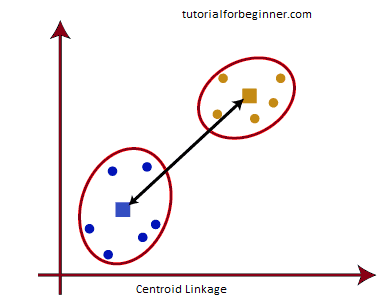From the above-given approaches, we can apply any of them according to the type of problem or business requirement.

## Woking of Dendrogram in Hierarchical clustering

The dendrogram is a tree-like structure that is primarily used to store each HC algorithm step as a memory. The Y-axis in the dendrogram display represents the Euclidean distances between the data points, while the x-axis represents the total number of data points in the dataset.

The working of the dendrogram can be explained using the below diagram: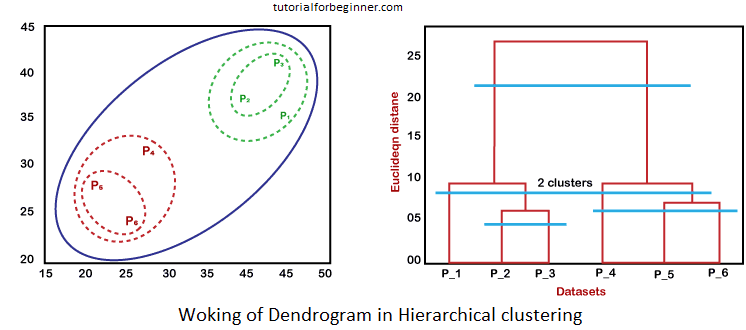The left side of the diagram depicts how clusters are formed in agglomerative clustering, while the right part depicts the matching dendrogram.

• As previously stated, the datapoints P2 and P3 join to form a cluster, and a dendrogram is formed as a result, which connects P2 and P3 in a rectangle shape. The height is determined by the distance between the data points in Euclidean space.
• P5 and P6 form a cluster in the next stage, and the associated dendrogram is formed. It's higher than before because the Euclidean distance between P5 and P6 is slightly longer than the distance between P2 and P3.
• Two new dendrograms are constructed this time, one combining P1, P2, and P3 and the other combining P4, P5, and P6.
• Finally, a final dendrogram is constructed that incorporates all of the data points.

According to our needs, we can cut the dendrogram tree structure at any level.

## Python Implementation of Agglomerative Hierarchical Clustering

Now we'll explore how to use Python to create the agglomerative hierarchical clustering technique. To do so, we'll utilize the same dataset problem we used in the preceding topic of K-means clustering, so we can simply compare the two concepts.

The dataset contains information about customers who have gone shopping at a mall. So, utilizing the dataset information, the mall owner wants to uncover certain patterns or specific customer behavior.

## Steps for implementation of AHC using Python:

The implementation procedures will be similar to those for k-means clustering, with a few exceptions, such as the mechanism for determining the number of clusters. The steps are as follows:

• Data Pre-processing
• Finding the optimal number of clusters using the Dendrogram
• Training the hierarchical clustering model
• Visualizing the clusters

## Data Pre-processing Steps:

In this step, we will import the libraries and datasets for our model.

• Importing the libraries
``````
#Importing the libraries
import numpy as nm
import matplotlib.pyplot as mtp
import pandas as pd
```
```

The above lines of code are used to import libraries that perform specific tasks, such as numpy for mathematical operations, matplotlib for graph or scatter plot drawing, and pandas for dataset import.

• Importing the dataset
``````
#Importing the dataset
```
```

As discussed above, we have imported the same dataset of Mall_Customers_data.csv, as we did in k-means clustering. Consider the below output: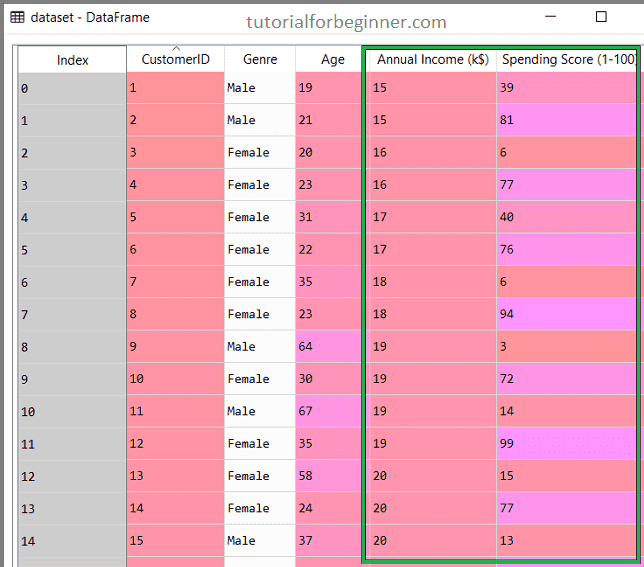• Extracting the matrix of features

We'll only extract the matrix of features because we don't know anything more about the dependent variable. The following is the code:

``````
x = dataset.iloc[:, [3, 4]].values
```
```

We've only retrieved 3 and 4 columns because we're going to use a 2D display to see the clusters. As a result, the Annual Income and Spending Score is used as a matrix of attributes.

## Step-2: Finding the optimal number of clusters using the Dendrogram

Now we'll use the Dendrogram to discover the best number of clusters for our model. We'll utilize the scipy library for this because it has a method that returns the dendrogram for our code directly. Consider the lines of code below:

``````
#Finding the optimal number of clusters using the dendrogram
import scipy.cluster.hierarchy as shc
mtp.title("Dendrogrma Plot")
mtp.ylabel("Euclidean Distances")
mtp.xlabel("Customers")
mtp.show()
```
```

The hierarchy module of the scipy library was imported in the above lines of code. This module includes the shc.denrogram() method, which accepts the linkage() parameter. We've supplied the x(matrix of features) and method "ward," a popular technique of linkage in hierarchical clustering, to the linkage function, which is used to establish the distance between two clusters.

The remaining lines of code are to describe the labels for the dendrogram plot.

### Output:

By executing the above lines of code, we will get the below output: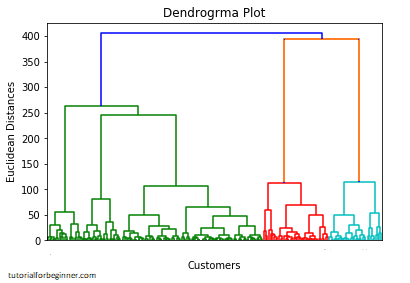We'll now use this Dendrogram to figure out how many clusters our model should include. We'll achieve this by determining the maximum vertical distance that avoids cutting any horizontal bars. Consider the diagram below: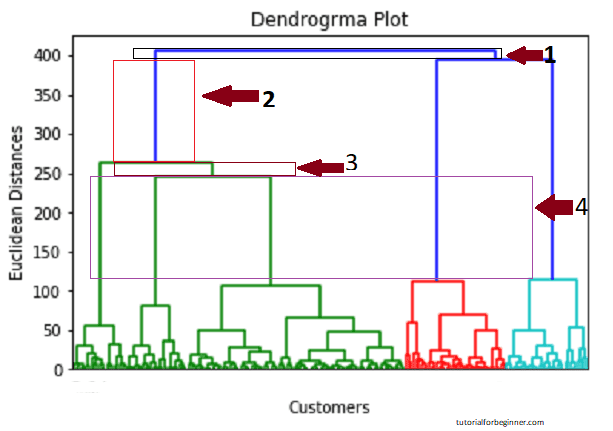The vertical lengths that do not cut their horizontal bars are indicated in the diagram above. As we can see, the fourth distance appears to be the greatest, hence the number of clusters will be five (the vertical lines in this range). We can also use the second number because it roughly equals the fourth distance, but we'll use the 5 clusters because that's what the K-means method calculated.

As a result, the best number of clusters is 5, and we'll use that number to train the model in the next stage.

## Step-3: Training the hierarchical clustering model

As we know the required optimal number of clusters, we can now train our model. The code is given below:

``````
#training the hierarchical model on dataset
from sklearn.cluster import AgglomerativeClustering
hc = AgglomerativeClustering(n_clusters = 5, affinity = 'euclidean', linkage = 'ward')
y_pred = hc.fit_predict(x)
```
```

The AgglomerativeClustering class of the cluster module of the scikit learn library is imported in the given code.

Then we created the hc object, which is a class object. The following parameters are passed to the AgglomerativeClustering class:

• n clusters=5: This specifies the number of clusters, and we've chosen 5 because it's the most optimal number.
• affinity='euclidean': It is a metric used to compute the linkage.
• linkage='ward': This specifies the linkage criterion; we've used the "ward" linkage in this case. This is the widely used linkage approach, which we have already used to create the Dendrogram. Each cluster's variance is reduced.

To fit or train the model, we created the dependent variable y_pred in the last line. Not only does it train the model, but it also returns the clusters that each data point belongs to.

We can inspect the y pred variable after executing the preceding lines of code using the variable explorer feature in our Sypder IDE. The original dataset can be compared to the y pred variable. Consider the following illustration: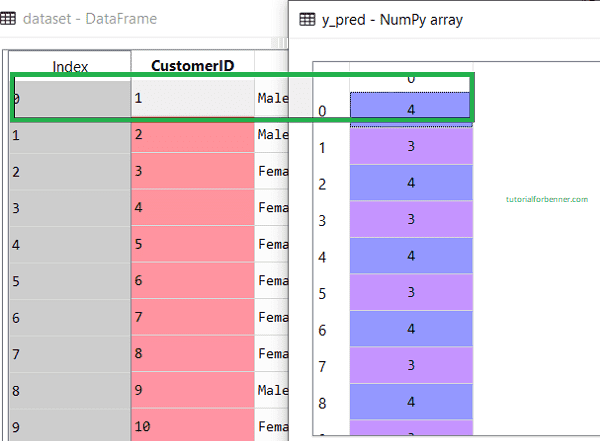As can be seen in the preceding image, the y_pred column displays the clusters value, indicating that customer id 1 belongs to the 5th cluster (indexing begins at 0), customer id 2 belongs to the 4th cluster, and so on.

## Step-4: Visualizing the clusters

Now that we've successfully trained our model, we can see the clusters that correlate to the dataset.

Except for one adjustment, we'll use the identical lines of code as we did for k-means clustering. Because we employed dendrogram to find the appropriate number of clusters, we will not plot the centroid as we did in k-means. The code is as follows:

``````
#visulaizing the clusters
mtp.scatter(x[y_pred == 0, 0], x[y_pred == 0, 1], s = 100, c = 'blue', label = 'Cluster 1')
mtp.scatter(x[y_pred == 1, 0], x[y_pred == 1, 1], s = 100, c = 'green', label = 'Cluster 2')
mtp.scatter(x[y_pred== 2, 0], x[y_pred == 2, 1], s = 100, c = 'red', label = 'Cluster 3')
mtp.scatter(x[y_pred == 3, 0], x[y_pred == 3, 1], s = 100, c = 'cyan', label = 'Cluster 4')
mtp.scatter(x[y_pred == 4, 0], x[y_pred == 4, 1], s = 100, c = 'magenta', label = 'Cluster 5')
mtp.title('Clusters of customers')
mtp.xlabel('Annual Income (k\$)')
mtp.ylabel('Spending Score (1-100)')
mtp.legend()
mtp.show()
```
```

Output: By executing the above lines of code, we will get the below output: